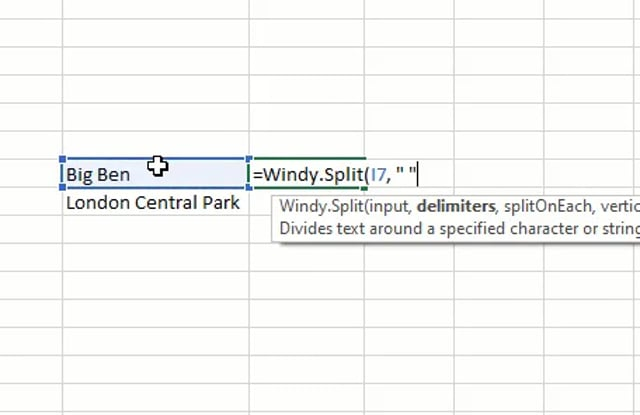# Windy.Geo

· Featured, Functions
A package containing Excel functions for working with geographical coordinates via the Google Geocoding API.

# Free#### Description

A collection of Excel functions for working with geographical coordinates:
• Windy.Geo.ParseLatitude
• Windy.Geo.ParseLongitude
• Windy.Geo.Distance
The Windy.Geo.GoogleGeocode and Windy.Geo.Distance functions require a Google API key to work, while Windy.Geo.ParseLatitude and Windy.Geo.ParseLongitude work on their own.#### Definitions and examples

##### Windy.Geo.ParseLatitude

Returns the latitude in decimal degrees from the given coordinates.

###### Examples:

`=Windy.Geo.ParseLatitude("41°24'12.2""N 2°10'26.5""E")`

`=Windy.Geo.ParseLatitude("41 24.2028, 2 10.4418")`

`=Windy.Geo.ParseLatitude("41.40338, 2.17403")`

A cell reference can also be an argument for the function.

##### Windy.Geo.ParseLongitude

Returns the longitude in decimal degrees from the given coordinates.

###### Examples:

`=Windy.Geo.ParseLongitude("41°24'12.2""N 2°10'26.5""E")`

`=Windy.Geo.ParseLongitude("41 24.2028, 2 10.4418")`

`=Windy.Geo.ParseLongitude("41.40338, 2.17403")`

A cell reference can also be an argument for the function.

Returns the geographical coordinates of an address/location.

###### Examples:

The following formula displays the coordinates of the Heathrow Airport in London.

`=Windy.Geo.GoogleGeocode("Heathrow Airport")`

A cell reference can also be an argument of the function.

##### Windy.Geo.Distance

Calculates and returns the distance between two points. The default unit is meters.

###### Examples:

The following formula calculates the distance between the two specified coordinates.

`=Windy.Geo.Distance("51.470023, -0.4542955","51.4993695, -0.1272993")`

`=Windy.Geo.Distance("51.470023, -0.4542955","51.4993695, -0.1272993", "mile")`

A cell reference can also be an argument of the function.